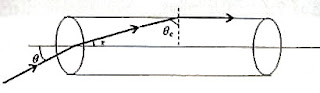## Wednesday, May 27, 2009

### AIEEE 2009 Multiple Choice Questions on Optics

I believe in standardising automobiles, not human beings

– Albert Einstein

The following three questions were included from optics in the All India Engineering/Architecture Entrance Examination (AIEEE) 2009 question paper:(1) A transparent solid cylindrical rod has a refractive index of 2/√3. It is surrounded by air. A light ray is incident at the mid point of one end of the rod as shown in the figure. The incident angle θ for which the light ray grazes along the wall of the rod is

(1) sin–1(1/√3)

(2) sin–1(1/2)

(3) sin–1(√3/2)

(4) sin–1(2/√3)

If n is the refractive index and θc is the critical angle of the material of the rod, we have n = 1/sinθc so that

sinθc = 1/n = √3/2.If r represents the angle of refracion (as shown in the figure), we have

1×sinθ = nsin r = (2/√3) ×sin(90º θc)

Or, sinθ = (2/√3) ×cos θc

But cos θc = ½ since sin θc = √3/2

[You may imagine a right angled triangle with opposite side √3 and hypotenuse 2. The adjacent side will be 1]

Substituting for cos θc, we obtain

sinθ = (2/√3) × ½ = 1/√3 so that θ = sin–1(1/√3)

(2) In an optics experiment, with the position of the object fixed, a student varies the position of a convex lens and for each position, the screen is adjusted to get a clear image of the object. A graph between the object distance u and the image distance v, from the lens, is plotted using the same scale for the two axes. A straight line passing through the origin and making an angle of 45° with the x-axis meets the experimental curve at P. The coordinates of P will be

(1) (4f, 4f)

(2) (2f, 2f)

(3) (f/2, f/2)

(4) (f, f)

This is a well known experiment which most of you might have performed. As such most of you know the answer: (2f, 2f)

We have 1/v – 1/u = 1/f

Applying the Cartesian sign convention, v is positive and u is negative so that

1/v + 1/u = 1/f

Since the straight line makes an angle of 45º, we have v = u at P and each must be equal to 2f to satisfy the equation, 1/v + 1/u = 1/f

(3) A mixture of light, consisting of wavelength 590 nm and an unknown wavelength, illuminates Young’s double slit and gives rise to two overlapping interference patterns on the screen. The central maximum of both lights coincide. Further, it is observed that the third bright fringe of known light coincides with the 4th bright fringe of the unknown light. From this data, the wavelength of the unknown light is

(1) 776.8 nm

(2) 393.4 nm

(3) 885.0 nm

(4) 442.5 nm

The fringe width β is given by

β = λD/d where λ is the wave length of light, D is the distance between the double slit and the screen and d is the double slit separation.

Since three fringes due to the light of wave length 590 nm exactly occupy the space occupied by four fringes due to the unknown light, we can write

3×590×D/d = 4λD/d where λ is the wave length of the unknown light (in nanometer).

This gives λ = 442.5 nm

You will find additional multiple choice questions (with solution) at the following locations:

1. apphysicsresources(i)